SLUSBH2G March   2013  – March 2019

PRODUCTION DATA.

1. Features
2. Applications
3. Description
1.     Device Images
4. Revision History
5. Pin Configuration and Functions
6. Specifications
7. Detailed Description
8. Application and Implementation
1. 8.1 Application Information
1. 8.1.1 Energy Harvester Selection
2. 8.1.2 Storage Element Selection
3. 8.1.3 Inductor Selection
4. 8.1.4 Capacitor Selection
2. 8.2 Typical Applications
1. 8.2.1 Solar Application Circuit
2. 8.2.2 TEG Application Circuit
3. 8.2.3 Piezoelectric Application Circuit
9. Power Supply Recommendations
10. 10Layout
11. 11Device and Documentation Support
12. 12Mechanical, Packaging, and Orderable Information

• RGR|20
• RGR|20

### 6.7 Typical Characteristics

Unless otherwise noted, graphs were taken using Figure 24 with CIN = 4.7µF, L1 = Coilcraft 22µH LPS4018, CSTOR = 4.7µF, L2 = Toko 10 µH DFE252012C, COUT = 22µF, VBAT_OV=4.2V, VOUT=1.8V

### Table 1. Table of Graphs

FIGURE
Charger Efficiency (η)(1) vs. Input Voltage IN= 10 µA Figure 1
IN= 100 µA Figure 2
IIN = 10 mA Figure 3
vs. Input Current VIN = 2.0 V Figure 4
VIN = 1.0 V Figure 5
VIN = 0.5 V Figure 6
VIN = 0.2 V Figure 7
VSTOR Quiescent Current vs. VSTOR Voltage EN = 1, VOUT_EN = X (Ship Mode) Figure 8
EN = 0, VOUT_EN = 0 (Standby Mode) Figure 9
VBAT Quiescent Current vs. VBAT Voltage EN = 0, VOUT_EN = 1 (Active Mode) Figure 10
Buck Efficiency (η) vs. Output Current Figure 11
vs. Input Voltage Figure 12
Normalized Buck Output Voltage vs. Output Current Figure 13
vs. Input Voltage Figure 14
vs. Temperature Figure 15
Buck Maximum Output Current vs. Input Voltage VOUT = 1.8V - 100mV Figure 16
Buck Major Switching Frequency vs. Output Current Figure 17
vs. Input Voltage Figure 18
Buck Output Ripple vs.Output Current Figure 19
vs. Input Voltage Figure 20
See SLUA691 for an explanation on how to take these measurements. Because the MPPT feature cannot be disabled on the bq25570, these measurements need to be taken in the middle of the 16 s sampling period.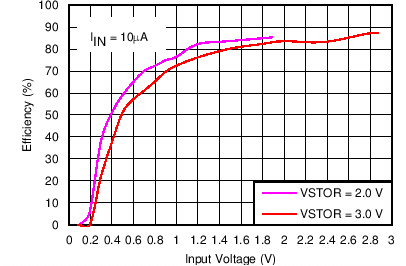VIN_DC = sourcemeter configured with ICOMP = 10 µA and outputing 0 to 3.0 V VSTOR = sourcemeter configured to measure current and voltage source set to hold the VSTOR voltage = 2.0 V or 3.0 V
Figure 1. Charger Efficiency vs Input VoltageVIN_DC = sourcemeter configured with ICOMP = 10 mA and voltage source varied from 0.1 V to 3.0 V VSTOR = sourcemeter configured to measure current and voltage source set to hold the VSTOR voltage = 2.0 V, 3.0 V or 5.5 V
Figure 3. Charger Efficiency vs Input Voltage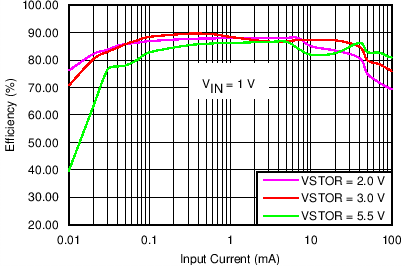VIN_DC = sourcemeter configured with voltage source = 1.0 V and ICOMP varied from 0.01 mA to 100 mA VSTOR = sourcemeter configured to measure current and voltage source set to hold the VSTOR voltage = 2.0 V, 3.0 V or 5.5 V
Figure 5. Charger Efficiency vs Input Current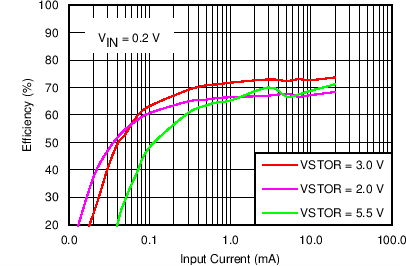VIN_DC = souremeter configured with voltage source = 0.2 V and ICOMP varied from 0.01 mA to 100 mA VSTOR = sourcemeter configured to measure current and voltage source set to hold the VSTOR voltage = 2.0 V, 3.0 V or 5.5 V
Figure 7. Charger Efficiency vs Input Current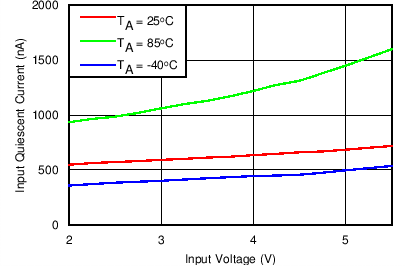VIN_DC = floating and EN = GND and VOUT_EN=VSTOR VSTOR= sourcemeter configured to measure current and voltage source varied from 2.0 V or 5.5 V
Figure 9. VSTOR Quiescent Current vs VSTOR Voltage: Active Mode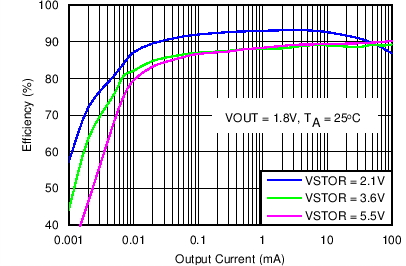VSTOR = sourcemeter configured as a voltage source, measuring current OUT = sourcemeter configured to sink current with VCOMP>V(OUT)
Figure 11. Buck Efficiency vs Output Current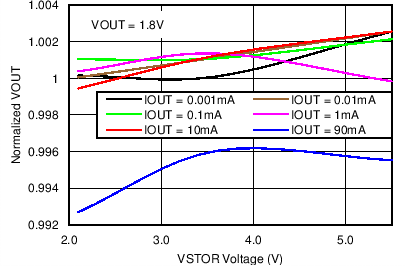VSTOR = sourcemeter configured as a voltage source OUT = sourcemeter configured to sink current with VCOMP>V(OUT) and measuring voltage
Figure 13. Normalized Buck Output Voltage vs Input Voltage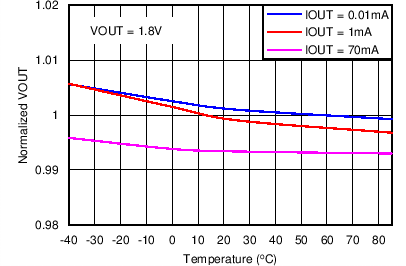VSTOR = sourcemeter configured as a voltage source OUT = sourcemeter configured to sink current with VCOMP>V(OUT) and measuring voltage Thermal stream for temperature variation
Figure 15. Normalized Buck Output Voltage vs Temperature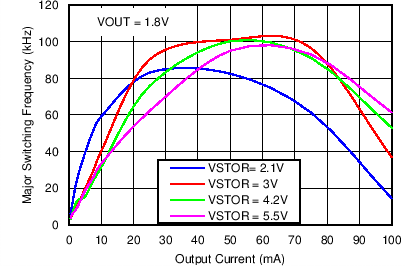VSTOR = sourcemeter configured as a voltage source OUT = sourcemeter configured to sink current with VCOMP>V(OUT) and measuring voltage Oscilloscope used to measure switching frequency at LBOOST
Figure 17. Buck Major Switching Frequency vs Output CurrentVSTOR = sourcemeter configured as a voltage source OUT = sourcemeter configured to sink current with VCOMP>V(OUT) and measuring voltage Oscilloscope used to measure voltage ripple at OUT
Figure 19. Buck Output Voltage Ripple vs Output Current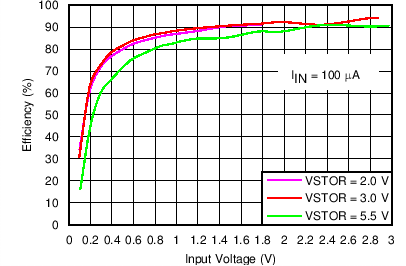VIN_DC = Keithley Source Meter configured with ICOMP = 100 µA and voltage source varied from 0.1 V to 3.0 V s VSTOR = Keithley Sourcemeter configured to measure current and voltage source set to hold the VSTOR voltage = 2.0 V, 3.0 V or 5.5 V
Figure 2. Charger Efficiency vs Input Voltage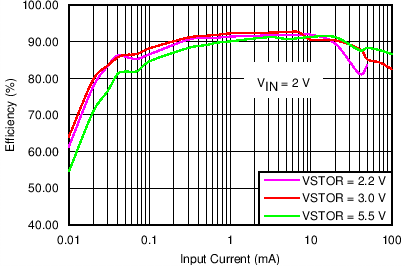VIN_DC = sourcemeter configured with voltage source = 2.0 V and ICOMP varied from 0.01 mA to 100 mA VSTOR = sourcemeter configured to measure current and voltage source set to hold the VSTOR voltage = 2.2 V , 3.0 V or 5.5 V
Figure 4. Charger Efficiency vs Input Current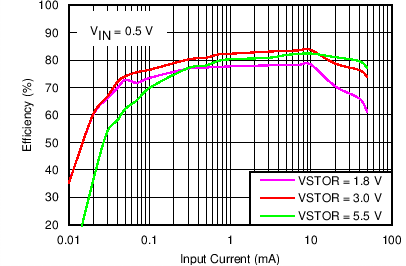VIN_DC = sourcemeter configured with voltage source = 0.5 V and ICOMP varied from 0.01 mA to 100 mA VSTOR = sourcemeter configured to measure current and voltage source set to hold the VSTOR voltage = 1.8 V, 3.0 V or 5.5 V
Figure 6. Charger Efficiency vs Input CurrentVIN_DC = floating and EN = VOUT_EN = GND VSTOR = sourcemeter configured to measure current and voltage source varied from 2.0 V or 5.5 V
Figure 8. VSTOR Quiescent Current vs VSTOR Voltage: Standby Mode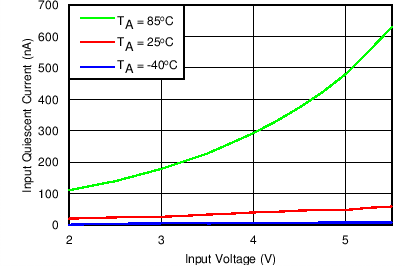VIN_DC = floating and EN = VSTOR VSTOR = sourcemeter configured to measure current and voltage source varied from 2.0 V or 5.5 V
Figure 10. VBAT Quiescent Current vs VBAT Voltage: Ship Mode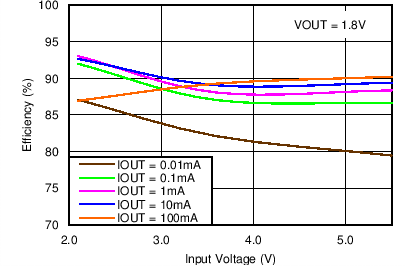VSTOR = sourcemeter configured as a voltage source, measuring current OUT = sourcemeter configured sink current with VCOMP>V(OUT)
Figure 12. Buck Efficiency vs Input Voltage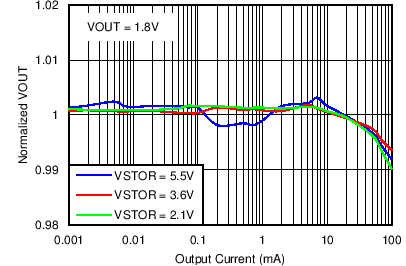VSTOR = sourcemeter configured as a voltage source OUT = sourcemeter configured to sink current with VCOMP>V(OUT) and measuring voltage
Figure 14. Normalized Buck Output Voltage vs Output Current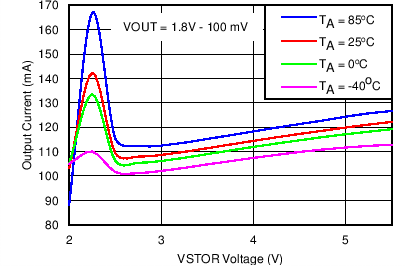VSTOR = sourcemeter configured as a voltage source OUT = sourcemeter configured to increasingly sink current with VCOMP>V(OUT) until V(OUT) < VOUT - 100 mV Thermal stream for temperature variation
Figure 16. Buck Maximum Output Current vs Input Voltage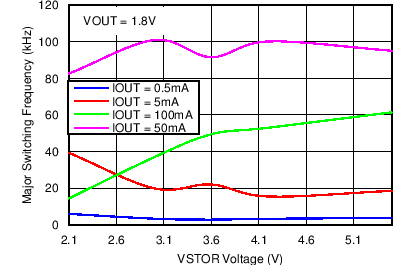VSTOR = sourcemeter configured as a voltage source OUT = sourcemeter configured to sink current with VCOMP>V(OUT) and measuring voltage Oscilloscope used to measure switching frequency at LBOOST
Figure 18. Buck Major Switching Frequency vs Input Voltage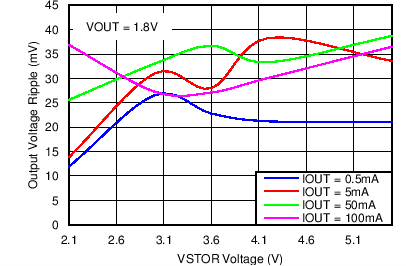VSTOR = sourcemeter configured as a voltage source OUT = sourcemeter configured to sink current with VCOMP>V(OUT) and measuring voltage Oscilloscope used to measure voltage ripple at OUT
Figure 20. Buck Output Voltage Ripple vs Input Voltage